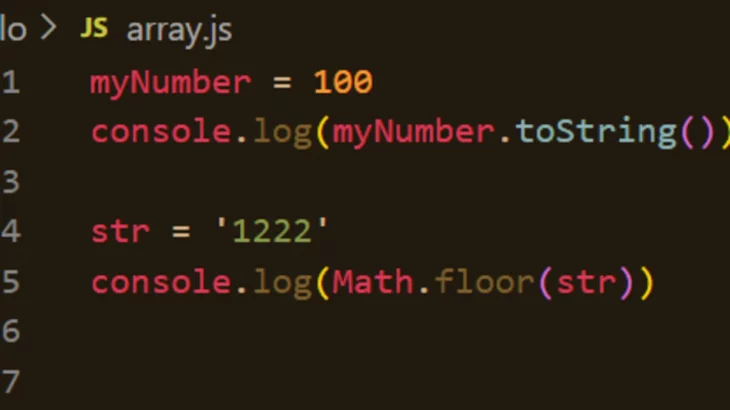# How to Check if String is Valid Number in JavaScript

Often JavaScript developers work with strings & numbers so it is always a good practice to check if a given string is a number or not. Using invalid numbers in JavaScript may result in error and terminate execution. This is required especially in form validation or even port number validation. In this article, we will learn how to check if string is valid number in JavaScript.

## How to Check if String is Valid Number in JavaScript

The simplest way to check if a string is a valid number or not is to use isNaN() function. The string NaN itself means ‘Not a Number’ so isNaN() function returns true if the input string is not a number, else it returns false.

`isNaN(num)         // returns true if the variable does NOT contain a valid number`

Here are some common use cases to call isNaN() with different types of input data.

```isNaN(123)         // false
isNaN('123')       // false
isNaN('1e10000')   // false (This translates to Infinity, which is a number)
isNaN('foo')       // true
isNaN('10px')      // true
isNaN('')          // false
isNaN(' ')         // false
isNaN(false)       // false```

As you can see above, isNaN() returns false even with empty strings and false boolean value. Other than that, it returns false for integers, floats, strings containing numbers and even numbers in exponential form.

The isNaN() is good enough for most websites and applications but isNaN() also returns false for empty strings. If you want a more stringent test for numbers here is a function you can use.

```function isNumeric(str) {
if (typeof str != "string") return false // we only process strings!
return !isNaN(str) && !isNaN(parseFloat(str))
}```

In the above function, we check if the input is a string, and if so we call parseFloat() function on it. parseFloat() function will return false for empty strings and strings of whitespace, unlike isNaN() so it handles that use case as well. Here is an example to illustrate this point.

```isNaN('')          // false
isNaN('   ')       // false

parseInt('')       // NaN
parseInt('   ')    // NaN```

On a separate note, if you want to convert a string to a number, you can simply prefix it with + operator.

```+num               // returns the numeric value of the string, or NaN
// if the string isn't purely numeric characters```

Here are some examples of the same.

```+'12'              // 12
+'12.'             // 12
+'12..'            // NaN
+'.12'             // 0.12
+'..12'            // NaN
+'foo'             // NaN
+'12px'            // NaN```

In this article, we have seen a couple of ways to check if string is valid number of not. You can use it in your form validation code, and even for validation of amounts, port number, etc.